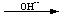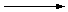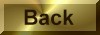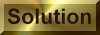Practice Problem 6

Write a balanced equation for the reaction between the permanganate ion and hydrogen peroxide in a basic solution to form manganese dioxide and oxygen.

 MnO4-(aq) + H2O2(aq)MnO2(s) + O2(g)

Answer

2 MnO4-(aq) + 3 H2O2(aq)2 MnO2(s) + 3 O2(g) + 2 OH-(aq) + 2 H2O(l)